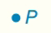Chapter 7.CT, Problem 4CT### Elementary Geometry for College St...

6th Edition
Daniel C. Alexander + 1 other
ISBN: 9781285195698

#### Solutions

Chapter
Section### Elementary Geometry for College St...

6th Edition
Daniel C. Alexander + 1 other
ISBN: 9781285195698
Textbook Problem
1 views

# Describe the locus of points in a plane that are at a distance of 3 cm from point P.______________________________________________________________________Exercises 4, 5

To determine

To describe:

The locus of points in a plane that are at a distance of 3 cm from point P.

Explanation

Definition:

Locus of points that are at a fixed distance r from fixed point P.

For example:

The circle with center P and radius r.

Calculation:

A locus is always an equal distance away from the given point...

### Still sussing out bartleby?

Check out a sample textbook solution.

See a sample solution

#### The Solution to Your Study Problems

Bartleby provides explanations to thousands of textbook problems written by our experts, many with advanced degrees!

Get Started

#### Convert the expressions in Exercises 6584 to power form. x23

Finite Mathematics and Applied Calculus (MindTap Course List)

#### 52. If

Mathematical Applications for the Management, Life, and Social Sciences

#### For y = (x3 + 1)2, y = _____. a) 2x(x3 + 1) b) 6x(x3 + 1) c) 6x2(x3 + 1) d) 3x2(x3 + 1)

Study Guide for Stewart's Single Variable Calculus: Early Transcendentals, 8th